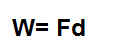﻿ Work Calculator

# Work CalculatorForce (F): N Distance (d) m

0.00J

This work calculator calculates the work done to an object, based on the amount of force, f, applied to the object and the distance, d, which the object moves due to this applied force, based on the formula shown above. So basically work is the amount of force needed to move an object a certain distance.

To use this calculator, a user just enters the force, f, applied to the object, measured in newtons (N), and the distance, d, that the object moves, measured in meters (m). The result of the work computation done is in unit joules (J).

A work calculator can be useful for electronics, especially when viewing it from a more physics perspective, because it can be used to calculate the work done on electrons in a circuit. The force that would be applied to electrons to make it to move would be a voltage source, because voltage gives electrons the push in order to move. This is why circuits need a power. The voltage is the power that which allows the electrons to move; and, in turn, this movement of electrons creates current. Current is electrons in motion. Thus, when we have this movement of electrons, or current, a device can be powered on. Thus, by knowing the force applied to objects such as electrons and the distance they move, we can compute the amount of work done in a circuit.

Related Resources